經濟學應用：無差異曲線 翁秉仁 ．作者任教於台大數學系 ．本文節錄改寫自作者《微積分講義》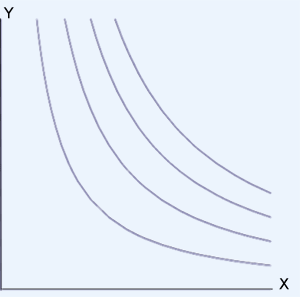X= 西瓜，Y= 冰淇淋

Claim.

(1) 遞減: 多吃西瓜就會少吃冰淇淋（這正是替代的意思）。
(2) 凹向上（即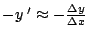會遞減）：當西瓜所佔的份量多時，多吃一片西瓜能代替的冰淇淋份量， 顯然比西瓜少時，多吃一片西瓜能代替的冰淇淋份量來得少，這是邊際效用遞減的結 果。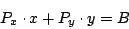f(x,y)=Px x+Py y-B=0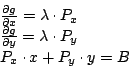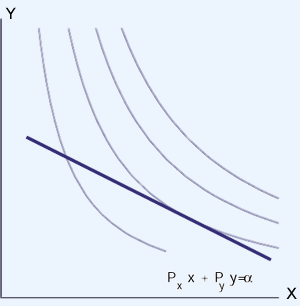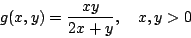Px=1, Py=2, B =9，代入前面的方程式得y=xy = -x 不合），所以 x+2y=9，解得 x=3, y=3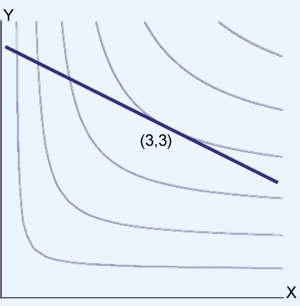(續上例) 若 Px=2, Py=1, 會造成偏袒嗎？

（Ans. 極值點條件為 y=2x，比例固定為 1:2，不因為預算升高而偏袒某商品。）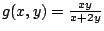, Px=1, Py=3, B=20

(1) 說明 g(x,y)=C>0 滿足無差異曲線的要求。

(2) 求極值點之條件。

(3) 有沒有因為預算升高而偏袒某商品的情形發生？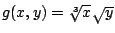, x, y>0, Px=1, Py=2, B =20.

(1) 說明 g(x,y)=C>0 滿足無差異曲線的要求。

(2) 求 x, y 購買之數量？

(3) 有沒有因為預算升高而偏袒某商品的情形發生？

(1) 說明 g(x,y)=C>0 滿足無差異曲線的要求。

(2) 取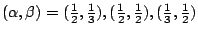三種情況，討論極值點條件與偏袒的問題。

(3) 證明當預算升高時，一般會發生如下偏袒現象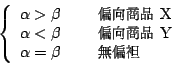（若有指正、疑問……，可以在此 留言 或 寫信 給我們。）EpisteMath (c) 2000 中央研究院數學所、台大數學系 各網頁文章內容之著作權為原著作人所有
 最後修改日期：9/30/2001